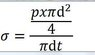# Strength of materials(longitudal stress in thin cylindrical shells

• oom kaaspaas

#### oom kaaspaas

In Rc Stephen's book Strength op materials, the longitudinal stress in a cylinder(see) attachment caputure.jpeg. My question is how is the area(pi X d X t ) is derived as my calculations show that this area should equate to (pi x d x t + t x t)

#### Attachments

•Capture.JPG
4.9 KB · Views: 893
the method used is the force projected on the circumference instead of the cross sectional area. From what I can gather this is wrong as the stress involved is Force dived by the cross sectional area.

I assume that d is the average diameter of the shell and t is its thickness.If the shell is thin (as in the title) then the area is approximately equal to pidt and I'm assuming that an approximate answer is good enough for this particular problem.

Thanks for your timely response and sorry for not spesifying. p is the atmospheric pressure,d is the inside diameter(thus excluding the thickness of the cylinder wall) and t is the thickness of the before mentioned wall. It is a valid point that as this is a thin cylinder the circumference rather than the wall area may be used. As such would using the wall area not be more accurate. if so what would such an equation be. My question is in regard to the stress created by a force tending to separate the left and right halves of the cylinder and as such is called longitudal stress as the direction of the stress is in the direction of the force. My second question is how was this formula pi x d x t derived

Last edited:
You stated the 'answer' to your own question in the thread title. Engineering is about making good assumptions. It's assumed that the cylinder is 'thin'.

In this case it's assumed that all the load is taken on the circuference, as you say you can use a thick walled solution (which modells the problem more accurately), it's more difficult to solve and the answers are likely to be similar. You will probably find a caveat in the book saying this is valid only when D > 10 or 20T

Say for example, that solving a full stress equation by hand takes you 10 minutes and you get an answer of 100. You could have applied an assumption and solved the problem in 5 minutes and get an answer of 95. Meaning the assumption loses you about 5% accuracy. Before doing this you need to ask yourself is the extra 5% accuracy really worth 100% extra calcualtion time.

The real area is equal to the area of the circle of diameter equal to d+2t minus the area of the circle of diameter equal to d and it would be more accurate to use the real area.If,however, t is small compared to d then the area is approximately equal to pidt and I assume from the title of the thread that it is acceptable to use this approximate value.

Thanks it makes sense, I still have two more questions on the subject namely how was piXDxT derived and can I use the same equation if there were a force tending to crush the cylinder asuming the t is reasonably smaller than d

Last edited:
I found the answer to my own question referring to how pi x D x T was derived. The full equation is pi x D x T + pi x T x T and as t is assumed to be small, T xT would be minute.
Now only the question if this equation holds for crushing stresses remains and I assume that it will.